Time: 3 Hours                                                                                                     Max. Marks: 100

NOTE: There are 9 Questions in all.

·      Question 1 is compulsory and carries 20 marks. Answer to Q. 1. must be written in the space provided for it in the answer book supplied and nowhere else.

·      Out of the remaining EIGHT Questions answer any FIVE Questions. Each question carries 16 marks.

·      Any required data not explicitly given, may be suitably assumed and stated.

Q.1       Choose the correct or best alternative in the following:                                         (2x10)

a.       The smallest positive integer n for which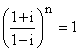is

(A)  8                                                  (B)  12

(C)    16                                               (D)  None of these

b.      A square root of 3 + 4i is

(A)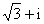(B)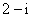(C)(D)  None of these

c.   Any vector a is equal to

(A)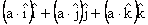(B)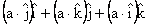(C)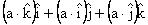(D)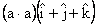d.   If a and b are two unit vectors inclined at an angle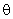and are such that a + b is a unit vector, thenis equal to

(A)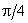(B)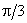(C)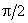(D)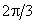e.   The value of the determinant, where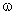is an imaginary cube root of unity is

(A)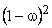(B)  3

(C)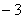(D)  4

f.    The value of the determineis equal to

(A)     -4                                                (B)  0

(C)  1                                                  (D)  4

g.   The inverse of a diagonal matrix is

(A)     not defined                                   (B)  a skew-symmetric matrix

(C)  a diagonal matrix                          (D) a unit matrix

h.   The period of function sin 2x + cot 3x + sec 5x is

(A)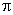(B)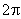(C)(D)i.    The Laplace transform of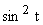is

(A)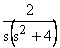(B)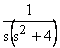(C)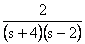(D)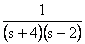j.    The solution of the differential equationis

(A)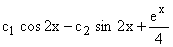(B)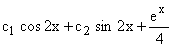(C)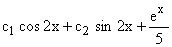(D)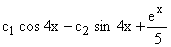Answer any FIVE Questions out of EIGHT Questions.

Each question carries 16 marks.

Q.2     a.   If n is a positive integer, prove that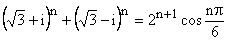.           (8)

b.   Find all the values of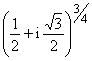and show that the product of all these values is 1.                   (8)

Q.3     a.   If the roots of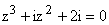represent vertices of a triangle in the Argand plane, then find area of the triangle.                                               (8)

b.   Find the value of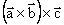if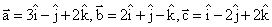.                                                    (8)

Q.4     a.   Prove that the sum of all the vectors drawn from the centre of a regular octagon to its vertices is the zero vector.                                                 (8)

b.   Find the moment about the point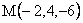of the force represented in magnitude and position by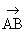, where the point A and B have the co-ordinates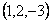and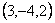respectively.           (8)

Q.5     a.   Show that.                                     (8)

b.   Write the following system of equations in the  matrix form AX = B and solve this for X by finding A-1.(8)

Q.6     a.   Using matrix methods, find the values of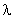and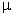so that the system of equations.

has (i) unique solution and (ii) has no solution                                                      (8)

b.   Verify Cayley Hamilton theorem for the matrix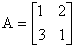.

Use Caley Hamilton theorem to evaluate A-1 and hence solve the equations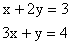(8)

Q.7           Find the Fourier series for the functions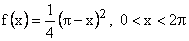(16)

Q.8       a.   Find the Laplace transform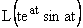(8)

b.   Find the inverse Laplace transform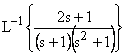(8)

Q.9       a.   Solve the differential equation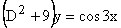(8)

b.   By using Laplace transform, solve the differential equation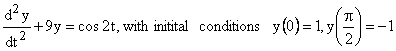(8)